output.to from Sideway
Number Theory

Fermat Number

Fermat Number

Draft for Information Only

# Content

` Fermat Numbers  Basic Properties of Fermat Numbers`

## Fermat Numbers

For a positive integer of the form 2p+1, the number can be a prime number, only if p is a power of 2. For a number of p with an odd factor, the number must be a composite number. Proof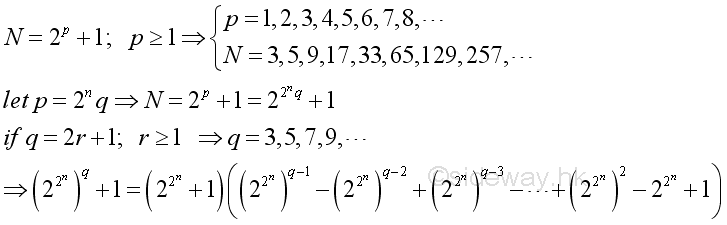Fermat studied these numbers and found that the first few numbers are prime numbers. Sometimes, but less common, a number of form ap=2p+1 is called Fermat number. But a Fermat number is usually refered to a number with the special case of the form Fn= 22n+1. Primes with this form are therefore called Fermat primes. For examples,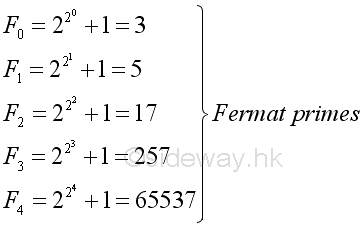However, Fermat numbers with n greater or equal to 5 are not always prime numbers. In fact, all known Fn as of 2015 are not prime numbers. For example, F5 is a composite.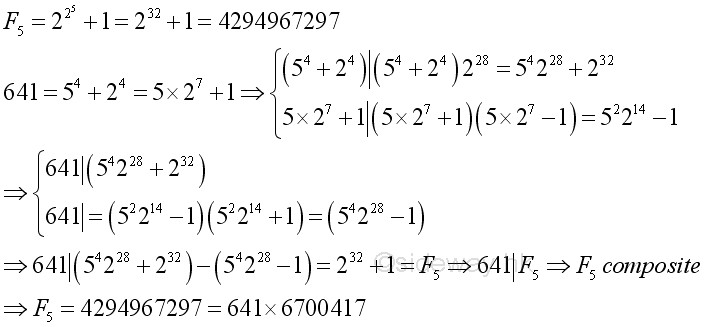### Basic Properties of Fermat Numbers

Basic properties or recurrence relations of Fermat Numbers

Proposition 1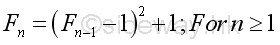Proof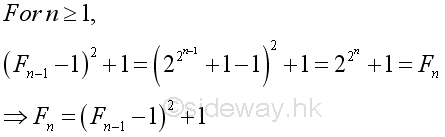Proposition 2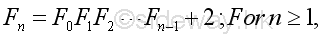Proof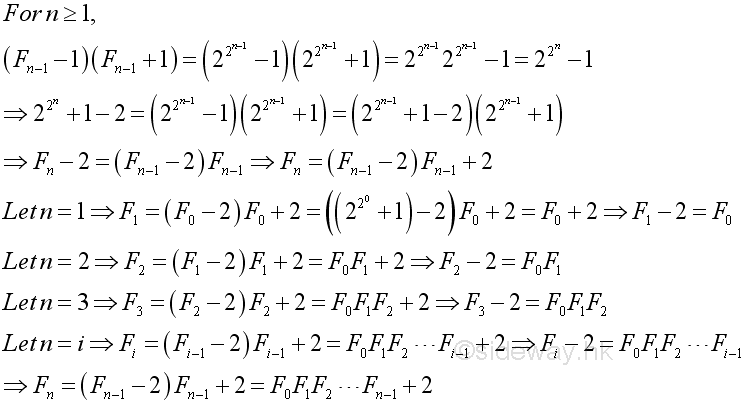• Corollary 2.1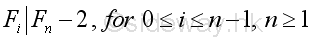Proof: Proposition 2 also implies that a Fermat number minus 2 is always divisible by all the smaller Fermat numbers for n greater than or equal to 1. Imply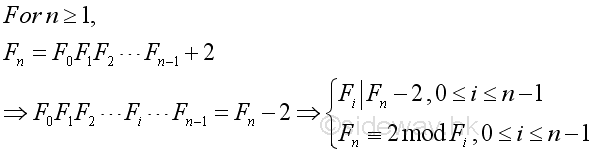• Corollary 2.2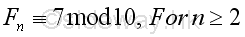Proof: For n greater than or equal to 2, Fn is greater than 5 and the last digit of Fn is always 7. Imply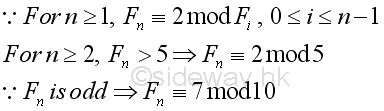• Corollary 2.3 No Fermat number is a perfect square.

Proof: For n=0, F0=3. For n=1, F1=5.  For n greater or equal 2, the last digit of Fn is always 7. Since a perfect square can only be congruent to 0, 1, 4, 5, 6, or 9 mod 10. Besides from Proposition 1, for n greater or equal 1, Fn is always equal to a perfect square number plus one, or Fn is always not equal to a perfect square.

• Corollary 2.4 For n greater than or equal to 1, Fermat number is of the form 6m-1.

Proof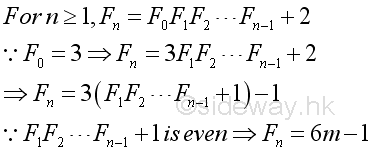Proposition 3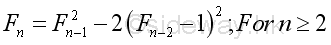Proof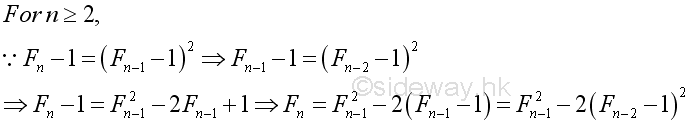Proposition 4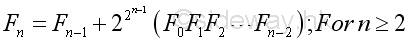Proof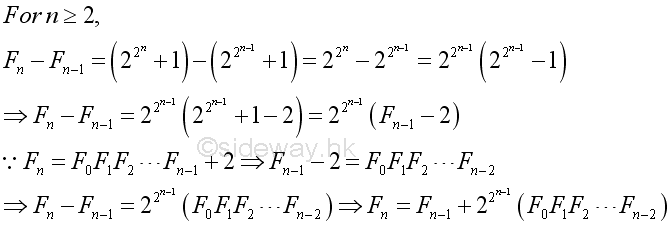Proposition 5   For n greater than or equal to 2, a Fermat number can has infinitely many representations of the form p2-2q2 where p and q are both positive integers.

Proof: Fermat numbers defined in Proposition 3 are of this form. In other words, for n great than or equal to 2, there always exists a pair of integers p and q. And other pairs of integer can be obtained recursively. Imply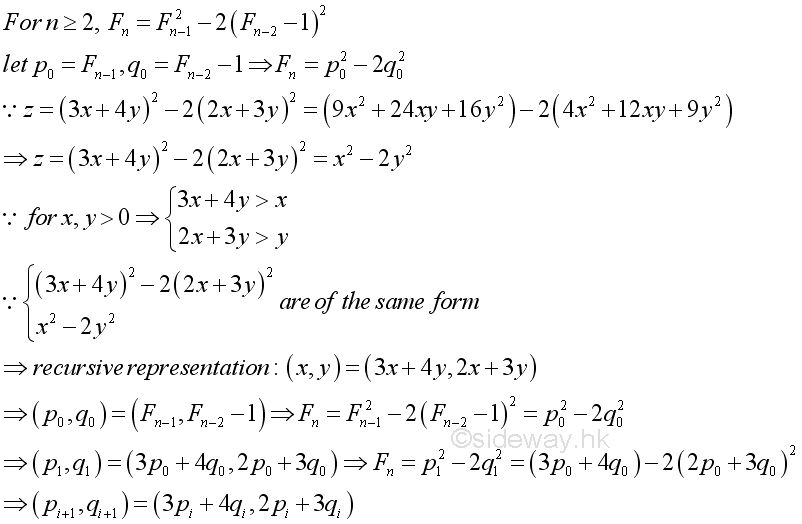Proposition 6   Any two Fermat numbers Fn=2 2n+1 are pairwise relatively prime to each other.

Proof: From Proposition 2, imply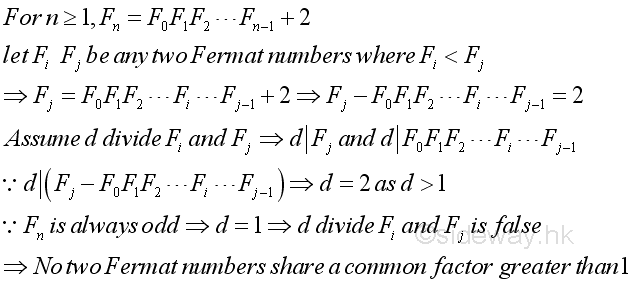• Corollary 6.1  There are infinitely many primes.

Proof: From Proposition  2, imply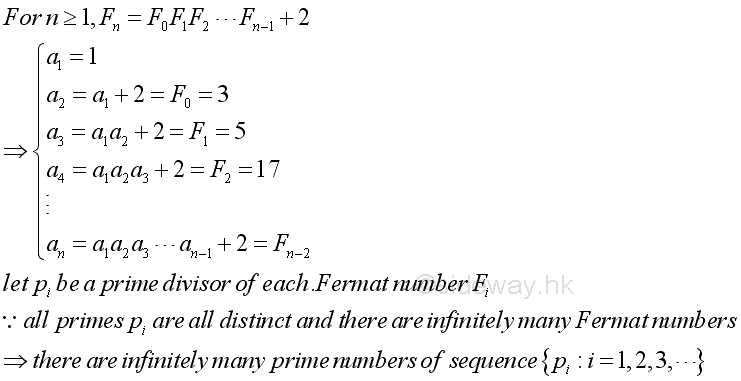Proposition 7   For n greater than or equal to 2, no Fermat number can be expressed in terms of the sum of two primes.

Proof: As in Proposition 2, imply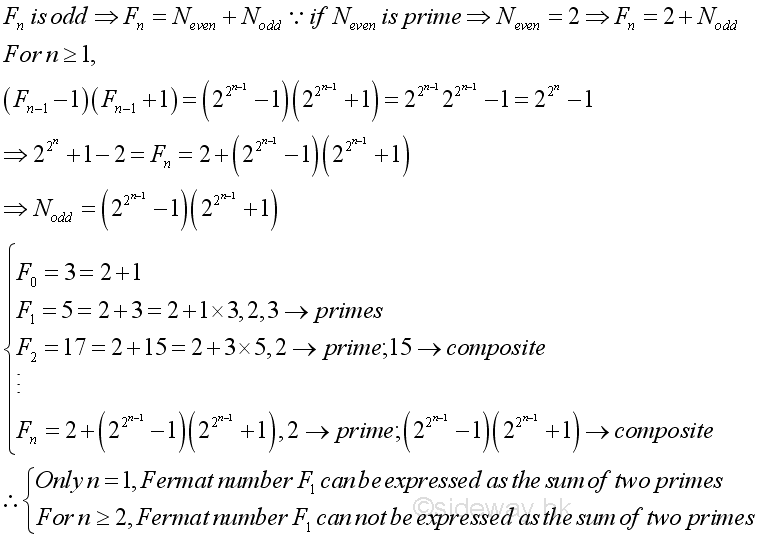©sideway

ID: 160100007 Last Updated: 2016/1/7 Revision:Home (5)

Business

Management

HBR (3)

Information

Recreation

Hobbies (7)

Culture

Chinese (1097)

English (336)

Reference (66)

Computer

Hardware (149)

Software

Application (187)

Digitization (24)

Numeric (19)

Programming

Web (644)CSS (SC)

ASP.NET (SC)

HTML

Knowledge Base

Common Color (SC)

Html 401 Special (SC)

OS (389)MS Windows

Windows10 (SC)

.NET Framework (SC)

DeskTop (7)

Knowledge

Mathematics

Formulas (8)

Number Theory (206)

Algebra (20)

Trigonometry (18)

Geometry (18)

Calculus (67)

Complex Analysis (21)

Engineering

Tables (8)

Mechanical

Mechanics (1)

Rigid Bodies

Statics (92)

Dynamics (37)

Fluid (5)

Control

Acoustics (19)

Biology (1)

Geography (1)

Latest Updated Links

Copyright © 2000-2019 Sideway . All rights reserved Disclaimers last modified on 10 Feb 2019